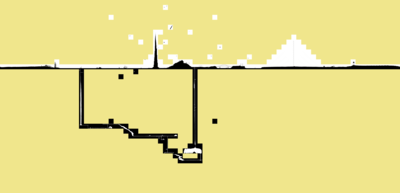# Map for xkcd No:1110

2012-09-21

Three times a week, Randall Munroe draws a new comic entry on xkcd.com. This Wednesday, the comic was quite unusual and pretty awesome: xkcd #1110. Basically, it's a giant map browsable with a limited field of view. I played with it for something like 12 seconds, and then decided to create a map so I wouldn't miss anything. Oh, just like pokanalysis...

This article is pretty accessible from a technical point of view, and I know a lot of other people did that as well, but I was willing to share the method I used anyway.

First, we need to grab all the images to process them locally.

We're going to use several scripts, so we need a place for some common code, let's say in a `xkcdcommon.py` script:

``````class XKCD1110:

def __init__(self):
self.size = [14, 48, 25, 33]
self.w = self.size + self.size
self.h = self.size + self.size

def tile_name(self, x, y):
x -= self.size
y -= self.size
return (('%d%c' % (y + 1, 's')) if y >= 0 else ('%d%c' % (-y, 'n'))) + \
(('%d%c' % (x + 1, 'e')) if x >= 0 else ('%d%c' % (-x, 'w')))
``````

This is a direct mapping of the code in 1110.js.

We now know the map has `width=48+33=81` and `height=14+25=39`. We also have a function to map the `[x;y]` position to the segmented image. Each image has a size of `2048x2048`. This is a pretty huge map we have there.

Since there is no listing of the images available, we will have to brute-force the download (if someone has another trick I'm interested). Here is the `img-url-list.py` script to print the full list of the potential images on stdout:

``````#!/usr/bin/env python

from xkcdcommon import XKCD1110

xkcd = XKCD1110()
for y in range(xkcd.h):
for x in range(xkcd.w):
print "http://imgs.xkcd.com/clickdrag/%s.png" % xkcd.tile_name(x, y)
``````

And we can download them in a `pic/` directory with the following `download-pic.sh` shell script:

``````#!/bin/sh

mkdir -p pic
for url in `./img-url-list.py`; do
wget -c "\$url" -P pic
sleep .5
done
``````

To simplify the reconstruction of the map, we might want to use a padding tile, let's generate one in a special color (to better mark the difference with the other tiles):

``````% convert -size 2048x2048 canvas:khaki pic/ref.png
``````

## Make the small tiles

The map is really large and we want to construct a preview, so we need a `reduce-images.sh` script to reduce each downloaded images in `pic/`:

``````#!/bin/sh

[ \$# -ne 1 ] && printf "usage: \$0 size\n" && exit 0

mkdir -p small
cd pic
for f in *.png; do
convert \$f -resize \${1}x\${1} ../small/\$f
done
``````

This will fill the `small/` directory with the reduced images (`2048x2048` to `64x64` if the run script parameter is `64`).

## Complete map image output

Now for our first output, we can construct a full picture of the reduced images using again ImageMagick. Here is a `output-image.py` python script to generate the appropriate `convert` command line to stack all the images into one:

``````#!/usr/bin/env python

import os.path
from xkcdcommon import XKCD1110

xkcd = XKCD1110()

cmd = 'convert'
for y in range(xkcd.h):
cmd += ' ('
for x in range(xkcd.w):
tile = 'small/' + xkcd.tile_name(x, y) + '.png'
if not os.path.isfile(tile):
tile = 'small/ref.png'
cmd += ' %s' % tile
cmd += ' +append )'
cmd += ' -append xkcd.png'
print(cmd)
``````

Pretty easy to use:

``````% \$(./output-image.py)
``````

And here is the `xkcd.png` output:## Complete map in HTML

Now it would be interesting to be able to zoom on a given part, so here is a `output-html.py` script to get a HTML output with clickable images:

``````#!/usr/bin/env python

import sys, os.path
from xkcdcommon import XKCD1110

xkcd = XKCD1110()

if len(sys.argv) != 2:
print('usage: %s size' % sys.argv)
sys.exit(0)

size = int(sys.argv)

<title>XKCD #1110 map</title>
for y in range(xkcd.h):
for x in range(xkcd.w):
tile = xkcd.tile_name(x, y) + '.png'
if not os.path.isfile('pic/' + tile):
tile = 'ref.png'
print('<a href="pic/%s" style="left:%dpx; top:%dpx;"><img src="small/%s" alt="" /></a>' % (tile, x*size, y*size, tile))
print('</body></html>')
``````

That script needs the sizes of the small pictures. Assuming we resized the images with `./resize-images.sh 64`, the HTML page can be generated with:

``````% ./output-html.py 64 > xkcd1110.html
``````

The output of the page is viewable here: interactive xkcd #1110 full map.

That's all folks.

For updates and more frequent content you can follow me on Mastodon. Feel also free to subscribe to the RSS in order to be notified of new write-ups. It is also usually possible to reach me through other means (check the footer below). Finally, discussions on some of the articles can sometimes be found on HackerNews, Lobste.rs and Reddit.